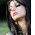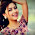## Tuesday, 23 February 2016

Introduction to Quantity comparison questions:

Each Quantitative Section in GRE contains about 7 Quantitative Comparison questions. As the name suggests ,  in these questions you need to compare two quantities mathematically and mark an answer accordingly. Also this a type of question not a topic , so you can get a quantity comparison question on any of the math topics( such as geometry , statistics etc ).

Since 35 percent of quantitative section constitutes of quantity comparison questions , it is very important to learn some strategies that can help students to solve these questions in lesser time and with better accuracy.

Format :

These questions have a very specific format : ‘column A’ and ‘column B’, each column containing numbers of variables. Often, you’ll get additional information about the variables. You will then have to choose one of the following 4 answers:
1. The quantity in column A is greater
2. The quantity in column B is greater
3. The two quantities are equal
4. The relationship cannot be determined from the information given

This might sound a bit confusing, but it’s pretty straightforward- all you need to do is analyze the information in both the columns and decide whether column A or B contains a larger quantity.Sometimes you are not able to compare the columns using given information or you end up getting more than one relation between the two columns , in that case D is your answer, and sometimes both the quantities are equal, so you’ll answer C. Check the following link to understand the questions in detail

Stratregies:

1. Know the format of questions and order of answer options

2. If you encounter a complex comparison than try and simplify that by applying the same operations to both columns. For example: you can multiply or divide both the columns by a same term or same number.

3. Focus on the information( if any) centred between the two columns.

4.  In case of variables in the questions consider what happens if you choose positive versus negative values, odd versus even values, and integer versus fractional values.

5.  ETS generally tests your knowledge of equations,  numbers  ,  geometry and arithmetic .

6.  Do plug in numbers into expressions with variables and if you get different results, than answer is D. Use FROZEN to choose numbers:

F- Fractions
R-Repeats ( Numbers present in  the questions)
O-One
Z-Zero
E-Extreme( Maximum and Minimum value a variable can take)
N-Negative

7.  Do not trust the geometric figures in comparison questions as they are never drawn to scale.

8.  Calculate to compare not to solve. Don’t do needless calculations . STOP when you get the comparison.

9.  Use FROZEN to consider all the possibilities in case of unknowns or variables.

10.  Don’t confuse percent and numbers.

11.  Estimate or Ballpark: Since there is no negative marking for an incorrect answer in GRE exam , it’s always better to ballpark and mark an answer.

12.  Use hints and mark Reasonable answers.

13.  Boundary condition helps us a lot

Quantity comparison questions are tricky and in order to score a 165+ in math section you need to avoid the TRAP answers. Also 14 out 40 scored questions would be quantity comparison questions so they are as important as any other type and require as much practice as Problem solving or Data interpretation questions.

Best of luck!!
www.wiseswan.com

#### 4 comments:

1.FROZEN,.. Nice and Useful suggestions.Take GRE Math Practice Test to analyze your abilities once in a week.

2.So now do we have to write GRE or only GRE subject test (Math) or both if we want to join a grad college or they are all just moot now ?

verbal gre portion

3.Someone essentially lend a hand to make severely posts I would state. That is the very first time I frequented your website page and thus far? I surprised with the analysis you made to create this particular submit incredible. Fantastic job!
Office Interior Designers in Coimbatore
Office Interior Designers in Bangalore
Office Interior Designers in Hyderabad

4.Usually I do not read post on blogs, but I would like to say that this write-up very forced me to try and do it! Your writing style has been surprised me. Thanks, very nice article.
SAP Training in Chennai
SAP Basis Training in Chennai
SAP SD Training in Chennai
SAP FICO Training in Chennai